# Selina Solutions Concise Mathematics Class 6 Chapter 5: Natural And Whole Numbers Exercise 5(F)

Selina Solutions Concise Mathematics Class 6 Chapter 5 Natural Numbers And Whole Numbers Exercise 5(F) gives a clear idea of solving the questions confidently. Practise is the key to scoring high marks in Mathematics. Thorough practice of Selina Solutions helps students in clearing their doubts, along with boosting exam preparation. The main purpose of creating solutions by experts at BYJU’S is to help students build a strong foundation in the subjects. For further detail, students can refer to Selina Solutions Concise Mathematics Class 6 Chapter 5 Natural Numbers And Whole Numbers Exercise 5(F) PDF, for free download from the links available below.

## Selina Solutions Concise Mathematics Class 6 Chapter 5: Natural Numbers And Whole Numbers Exercise 5(F) Download PDF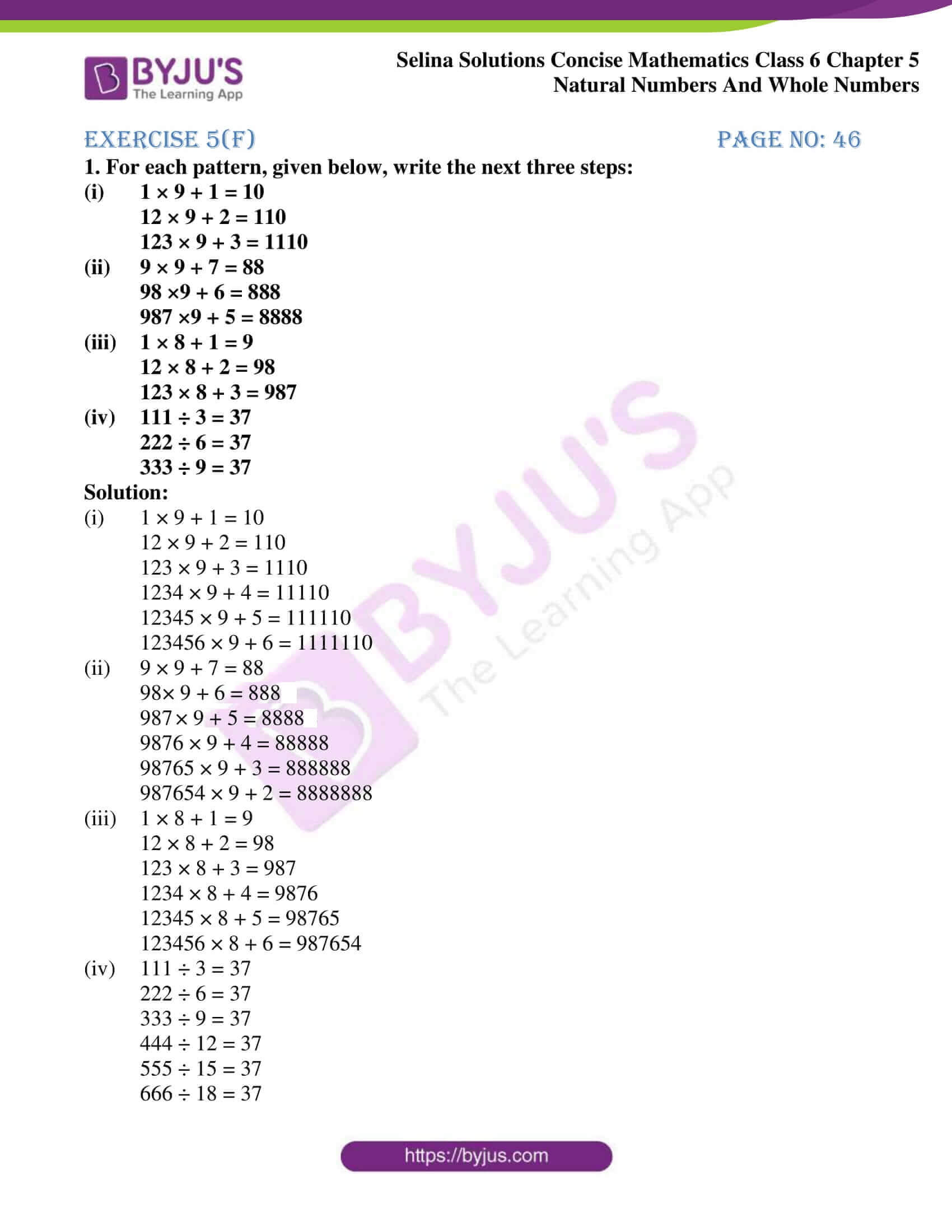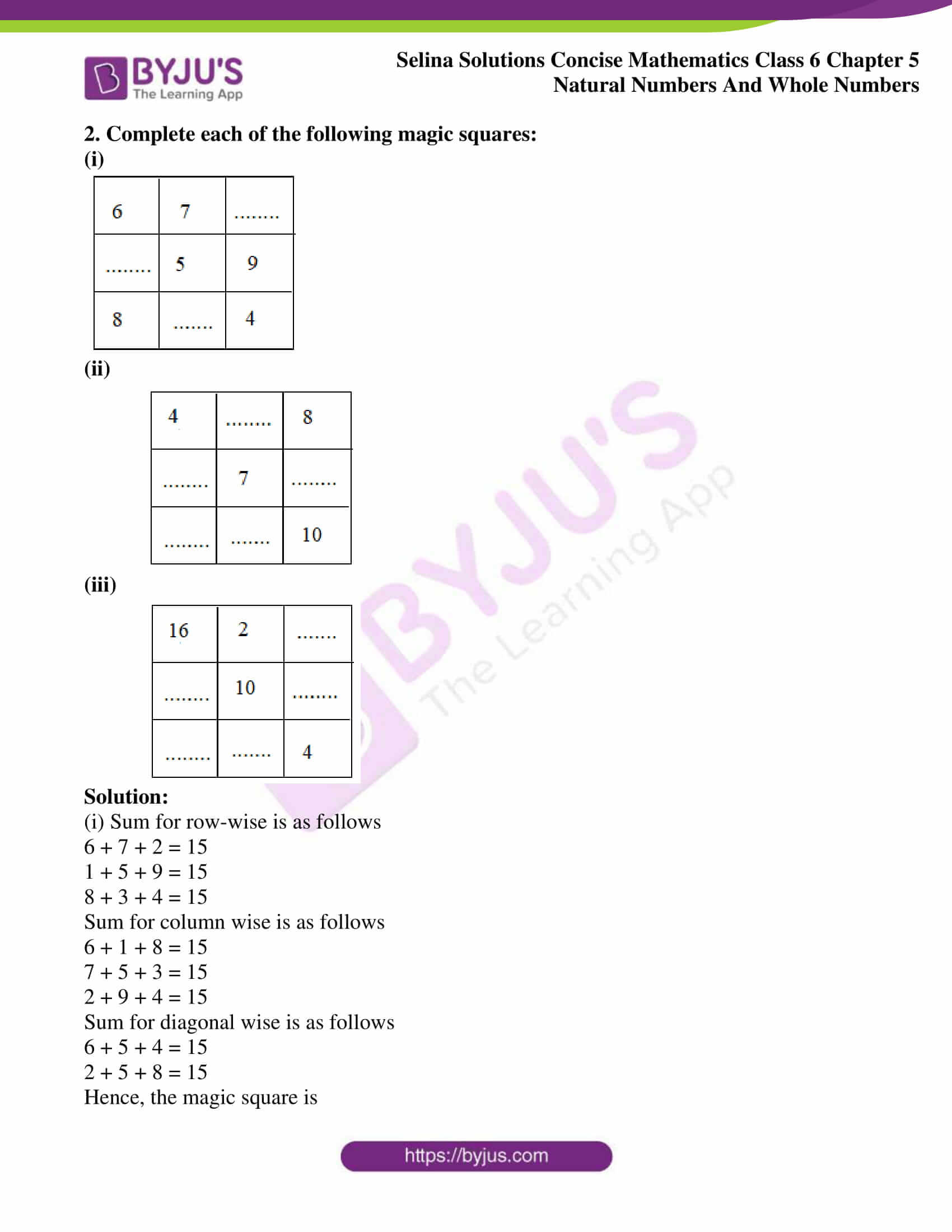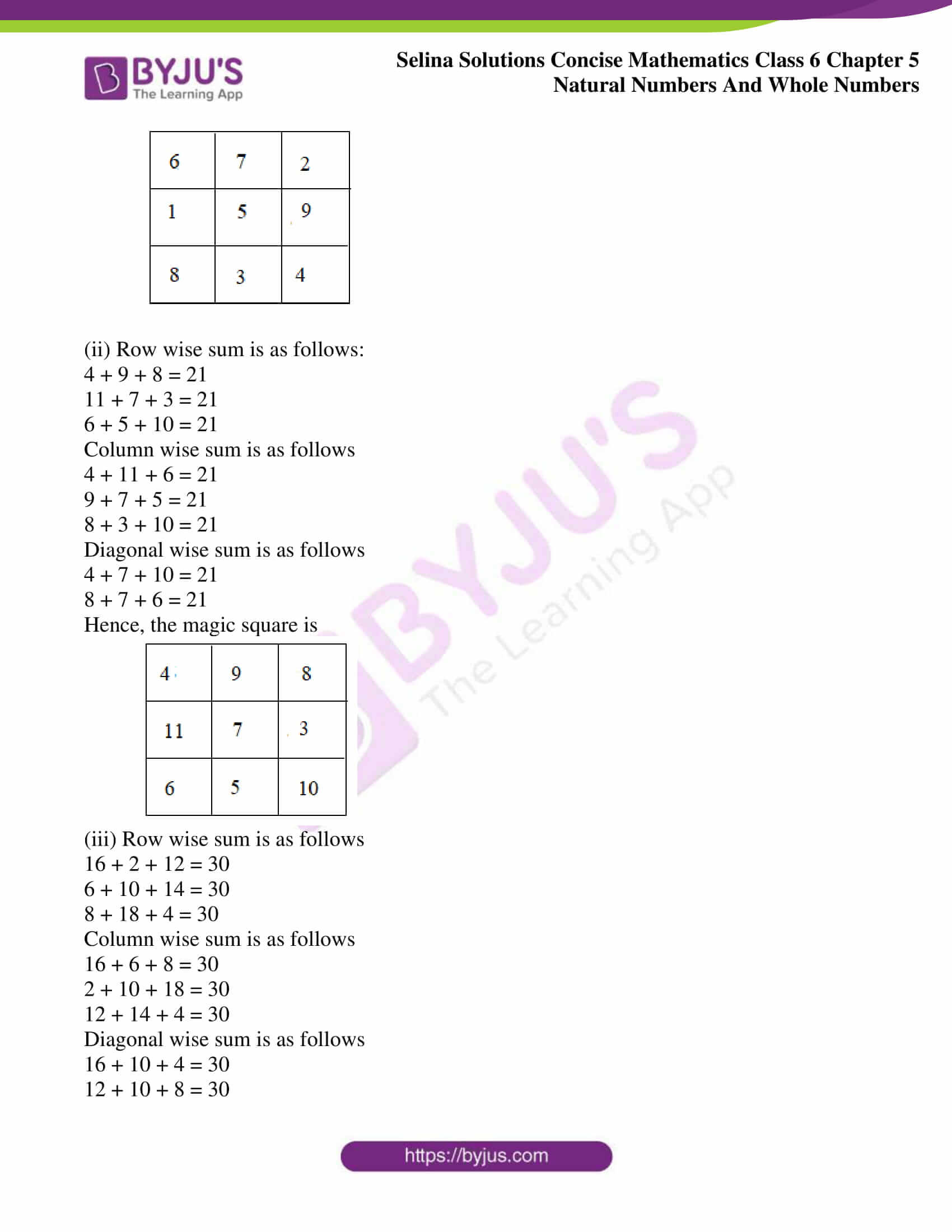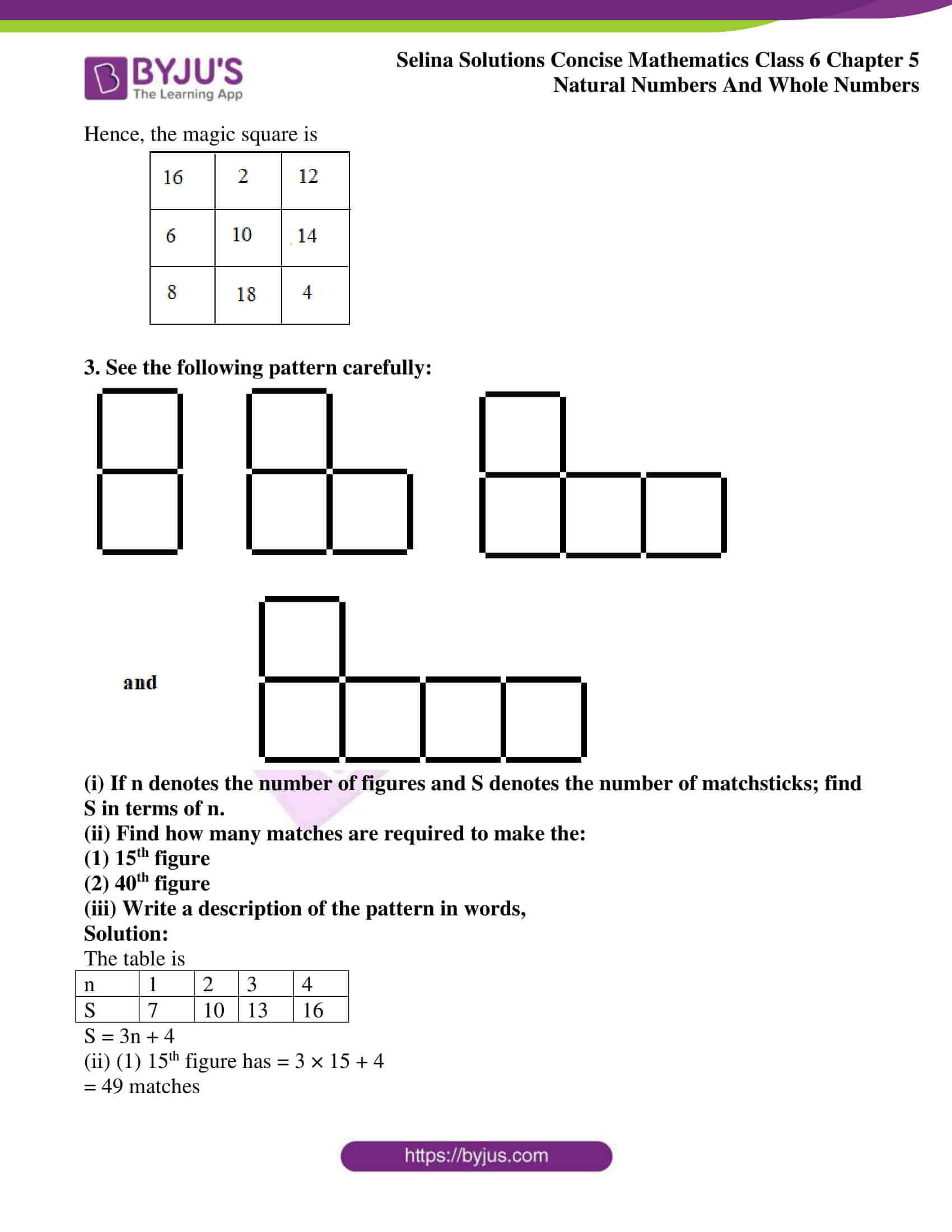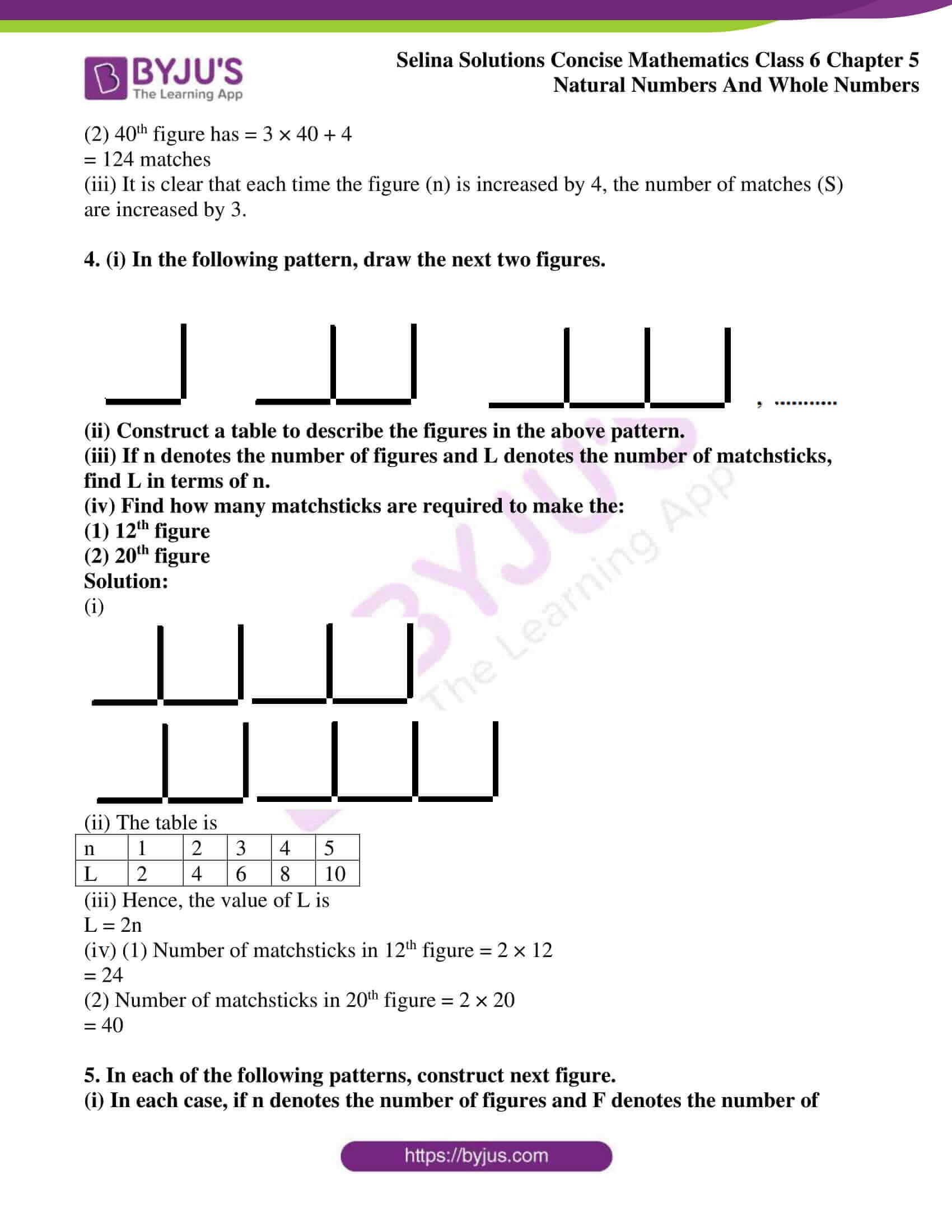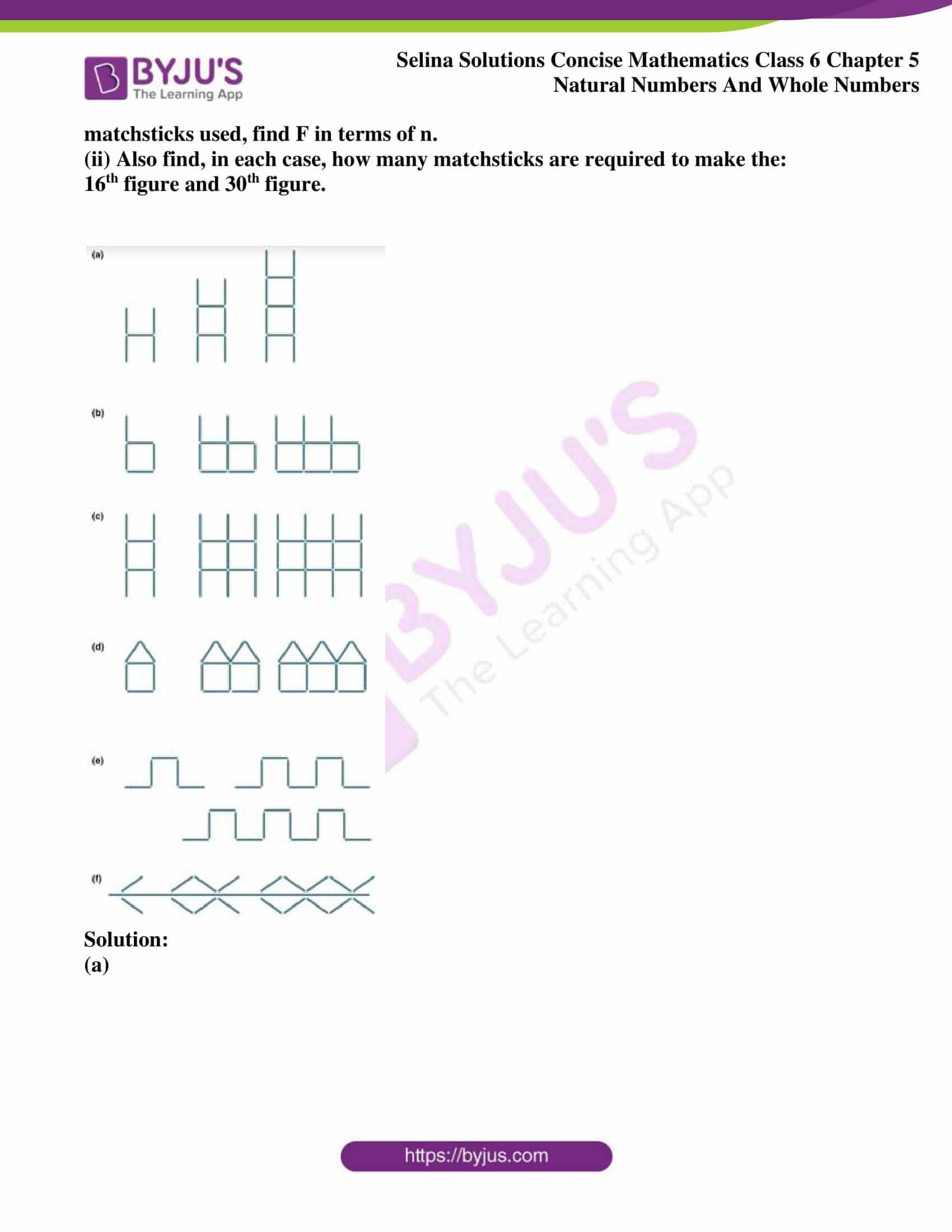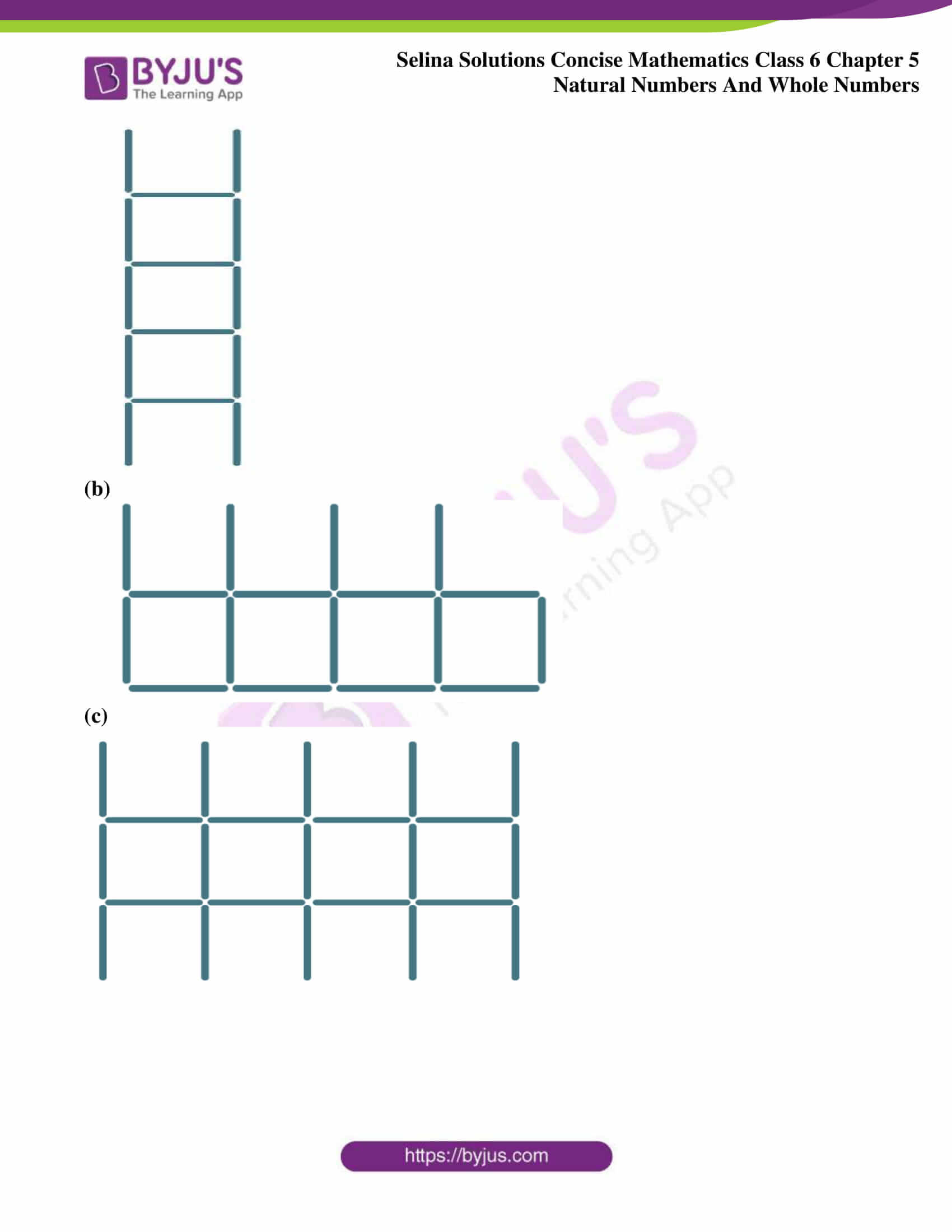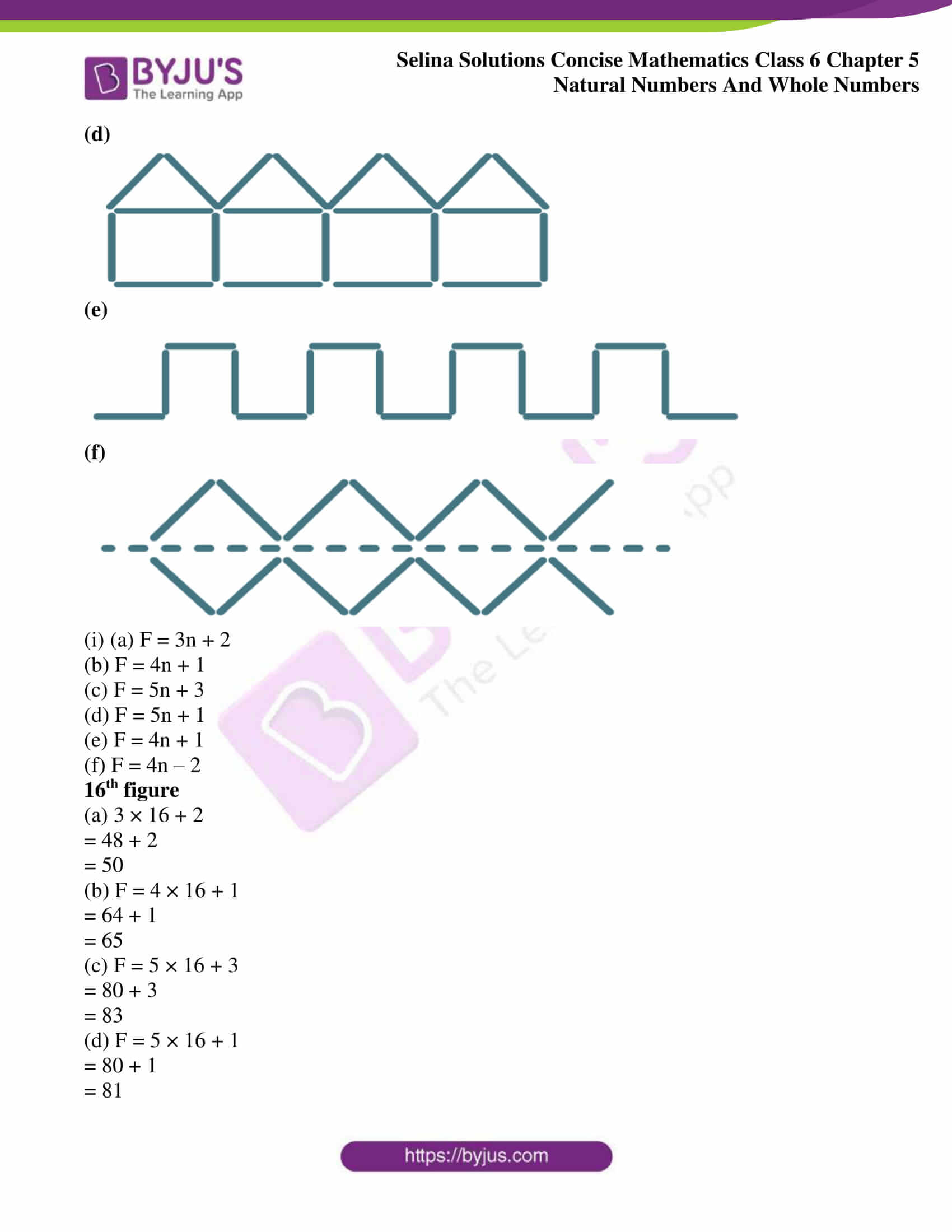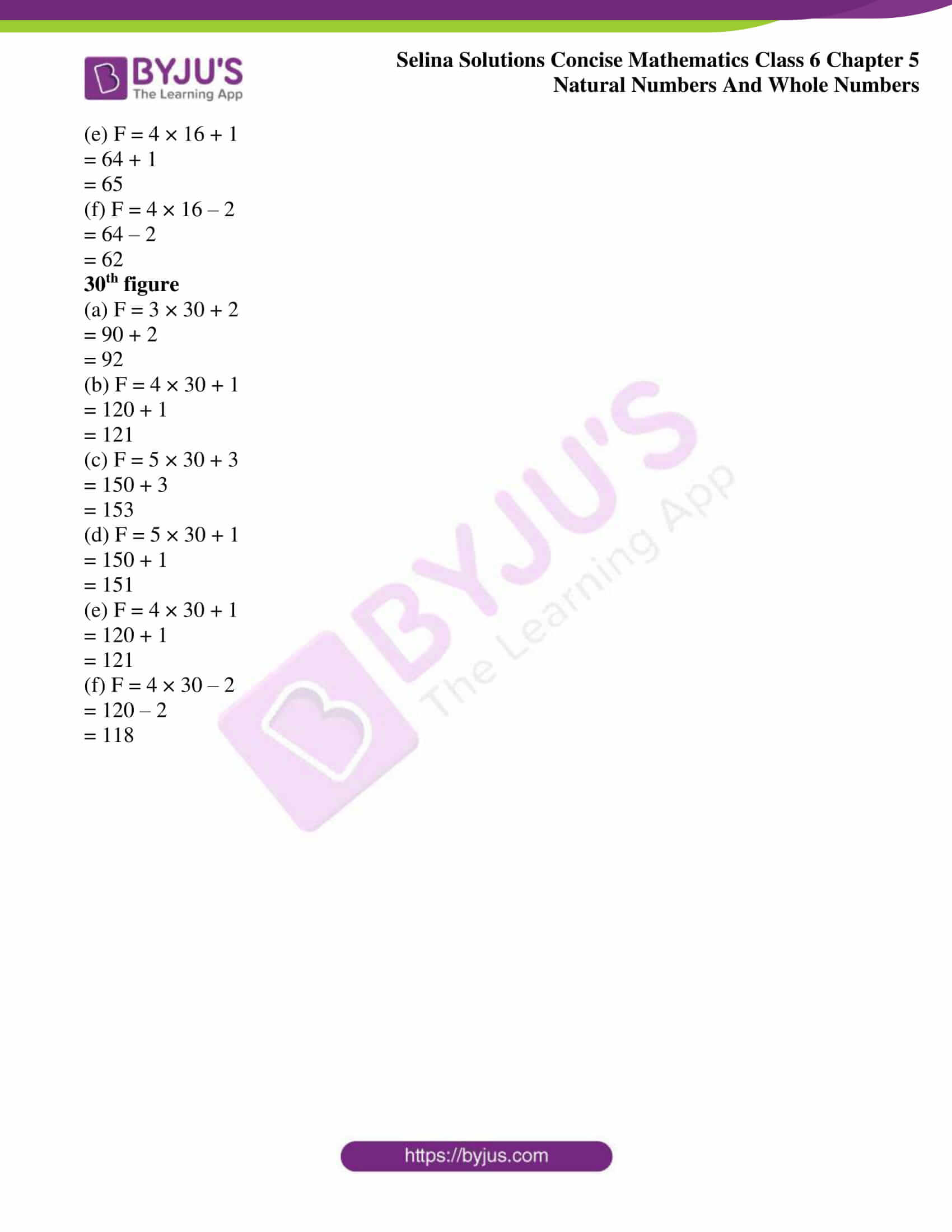### Access other exercises of Selina Solutions Concise Mathematics Class 6 Chapter 5: Natural Numbers And Whole Numbers

Exercise 5(A) Solutions

Exercise 5(B) Solutions

Exercise 5(C) Solutions

Exercise 5(D) Solutions

Exercise 5(E) Solutions

### Access Selina Solutions Concise Mathematics Class 6 Chapter 5: Natural Numbers And Whole Numbers Exercise 5(F)

#### Exercise 5(F) page no: 46

1. For each pattern, given below, write the next three steps:

(i) 1 × 9 + 1 = 10

12 × 9 + 2 = 110

123 × 9 + 3 = 1110

(ii) 9 × 9 + 7 = 88

98 ×9 + 6 = 888

987 ×9 + 5 = 8888

(iii) 1 × 8 + 1 = 9

12 × 8 + 2 = 98

123 × 8 + 3 = 987

(iv) 111 ÷ 3 = 37

222 ÷ 6 = 37

333 ÷ 9 = 37

Solution:

(i) 1 × 9 + 1 = 10

12 × 9 + 2 = 110

123 × 9 + 3 = 1110

1234 × 9 + 4 = 11110

12345 × 9 + 5 = 111110

123456 × 9 + 6 = 1111110

(ii) 9 × 9 + 7 = 88

98 × 9 + 6 = 888

987 × 9 + 5 = 8888

9876 × 9 + 4 = 88888

98765 × 9 + 3 = 888888

987654 × 9 + 2 = 8888888

(iii) 1 × 8 + 1 = 9

12 × 8 + 2 = 98

123 × 8 + 3 = 987

1234 × 8 + 4 = 9876

12345 × 8 + 5 = 98765

123456 × 8 + 6 = 987654

(iv) 111 ÷ 3 = 37

222 ÷ 6 = 37

333 ÷ 9 = 37

444 ÷ 12 = 37

555 ÷ 15 = 37

666 ÷ 18 = 37

2. Complete each of the following magic squares:

(i)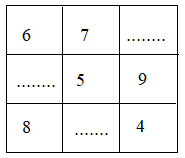(ii)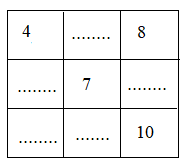(iii)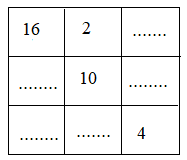Solution:

(i) Sum for row-wise is as follows

6 + 7 + 2 = 15

1 + 5 + 9 = 15

8 + 3 + 4 = 15

Sum for column wise is as follows

6 + 1 + 8 = 15

7 + 5 + 3 = 15

2 + 9 + 4 = 15

Sum for diagonal wise is as follows

6 + 5 + 4 = 15

2 + 5 + 8 = 15

Hence, the magic square is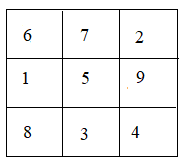(ii) Row wise sum is as follows:

4 + 9 + 8 = 21

11 + 7 + 3 = 21

6 + 5 + 10 = 21

Column wise sum is as follows

4 + 11 + 6 = 21

9 + 7 + 5 = 21

8 + 3 + 10 = 21

Diagonal wise sum is as follows

4 + 7 + 10 = 21

8 + 7 + 6 = 21

Hence, the magic square is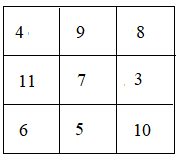(iii) Row wise sum is as follows

16 + 2 + 12 = 30

6 + 10 + 14 = 30

8 + 18 + 4 = 30

Column wise sum is as follows

16 + 6 + 8 = 30

2 + 10 + 18 = 30

12 + 14 + 4 = 30

Diagonal wise sum is as follows

16 + 10 + 4 = 30

12 + 10 + 8 = 30

Hence, the magic square is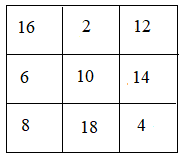3. See the following pattern carefully: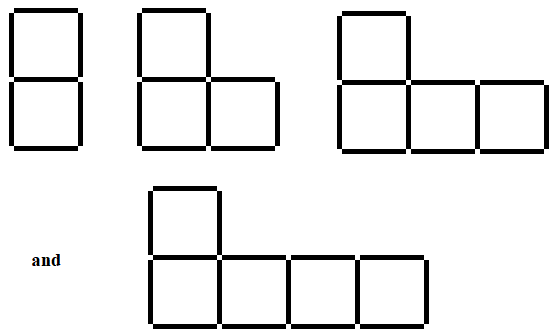(i) If n denotes the number of figures and S denotes the number of matchsticks; find S in terms of n.

(ii) Find how many matches are required to make the:

(1) 15th figure

(2) 40th figure

(iii) Write a description of the pattern in words,

Solution:

The table is

 n 1 2 3 4 S 7 10 13 16

S = 3n + 4

(ii) (1) 15th figure has = 3 × 15 + 4

= 49 matches

(2) 40th figure has = 3 × 40 + 4

= 124 matches

(iii) It is clear that each time the figure (n) is increased by 4, the number of matches (S) are increased by 3.

4. (i) In the following pattern, draw the next two figures.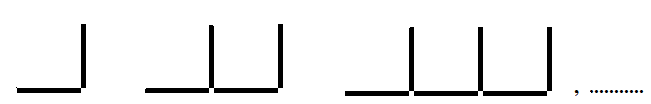(ii) Construct a table to describe the figures in the above pattern.

(iii) If n denotes the number of figures and L denotes the number of matchsticks, find L in terms of n.

(iv) Find how many matchsticks are required to make the:

(1) 12th figure

(2) 20th figure

Solution:

(i)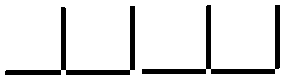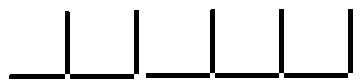(ii) The table is

 n 1 2 3 4 5 L 2 4 6 8 10

(iii) Hence, the value of L is

L = 2n

(iv) (1) Number of matchsticks in 12th figure = 2 × 12

= 24

(2) Number of matchsticks in 20th figure = 2 × 20

= 40

5. In each of the following patterns, construct next figure.

(i) In each case, if n denotes the number of figures and F denotes the number of matchsticks used, find F in terms of n.

(ii) Also find, in each case, how many matchsticks are required to make the:

16th figure and 30th figure.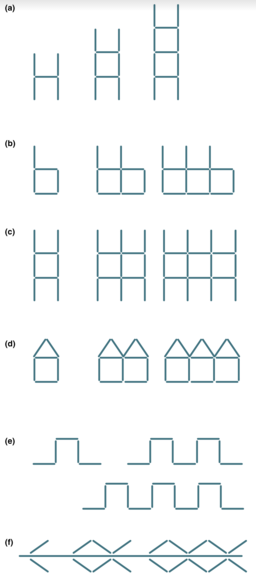Solution:

(a)(b)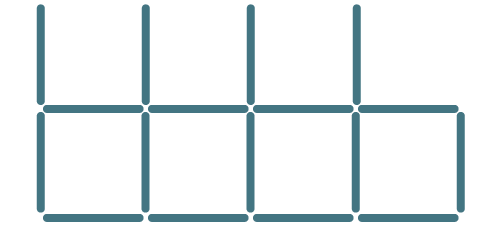(c)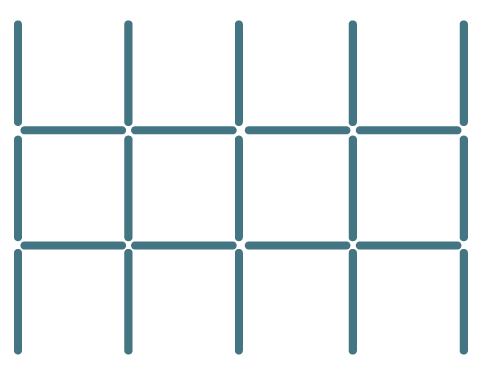(d)(e)(f)(i) (a) F = 3n + 2

(b) F = 4n + 1

(c) F = 5n + 3

(d) F = 5n + 1

(e) F = 4n + 1

(f) F = 4n – 2

16th figure

(a) 3 × 16 + 2

= 48 + 2

= 50

(b) F = 4 × 16 + 1

= 64 + 1

= 65

(c) F = 5 × 16 + 3

= 80 + 3

= 83

(d) F = 5 × 16 + 1

= 80 + 1

= 81

(e) F = 4 × 16 + 1

= 64 + 1

= 65

(f) F = 4 × 16 – 2

= 64 – 2

= 62

30th figure

(a) F = 3 × 30 + 2

= 90 + 2

= 92

(b) F = 4 × 30 + 1

= 120 + 1

= 121

(c) F = 5 × 30 + 3

= 150 + 3

= 153

(d) F = 5 × 30 + 1

= 150 + 1

= 151

(e) F = 4 × 30 + 1

= 120 + 1

= 121

(f) F = 4 × 30 – 2

= 120 – 2

= 118